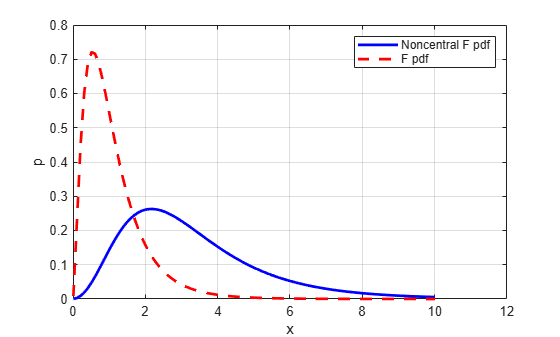# ncfpdf

Noncentral F probability density function

## Syntax

```Y = ncfpdf(X,NU1,NU2,DELTA) ```

## Description

`Y = ncfpdf(X,NU1,NU2,DELTA)` computes the noncentral F pdf at each of the values in `X` using the corresponding numerator degrees of freedom in `NU1`, denominator degrees of freedom in `NU2`, and positive noncentrality parameters in `DELTA`. `X`, `NU1`, `N2`, and `B` can be vectors, matrices, or multidimensional arrays that all have the same size, which is also the size of `Y`. A scalar input for `P`, `NU1`, `NU2`, or `DELTA` is expanded to a constant array with the same dimensions as the other inputs.

The F distribution is a special case of the noncentral F where δ = 0. As δ increases, the distribution flattens like the plot in the example.

## Examples

collapse all

Compute the pdf of a noncentral F distribution with degrees of freedom `NU1 = 5` and `NU2 = 20`, and noncentrality parameter `DELTA = 10`. For comparison, also compute the pdf of an F distribution with the same degrees of freedom.

```x = (0.01:0.1:10.01)'; p1 = ncfpdf(x,5,20,10); p = fpdf(x,5,20);```

Plot the pdf of the noncentral F distribution and the pdf of the F distribution on the same figure.

```figure; plot(x,p1,'b-','LineWidth',2) hold on plot(x,p,'g--','LineWidth',2) legend('Noncentral F','F distribution')```## References

 Johnson, N., and S. Kotz. Distributions in Statistics: Continuous Univariate Distributions-2. Hoboken, NJ: John Wiley & Sons, Inc., 1970, pp. 189–200.

## Version History

Introduced before R2006a